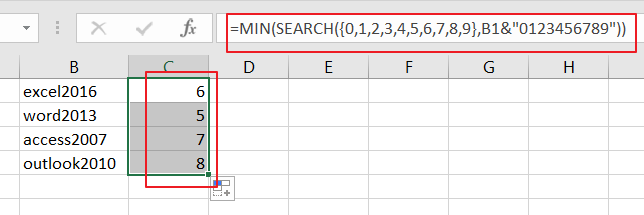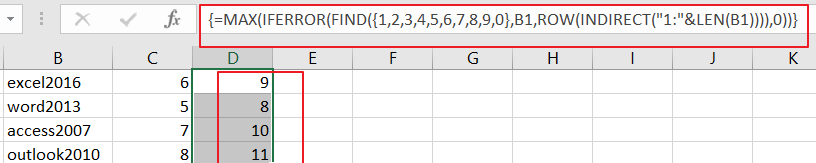# How to Find the Position of First Number in A Text String in Excel

This post will guide you how to get the position of the first number in a text string in Excel. How do I find the position of first number in a text string in Excel 2013/2016.

## Find the Position of First Number in a Cell

Assuming that you have a list of data in range B1:B4, in which contain text strings. And you wish to get the position of the first number in those range of cells. How to accomplish it. And you can use an formula based on the MIN function and the SEARCH function. Like below:

`=MIN(SEARCH({0,1,2,3,4,5,6,7,8,9},B1&"0123456789"))`

Type this formula into a blank cell and press Enter key on your keyboard, and drag the AutoFill Handle down to other cells to apply this formula.You would see that the position of the first number is calculated.

If you want to get the position of last number in a text string in your range of cells, you can use another array formula based on the MAX function, the IFERROR function, the FIND function, the ROW function, and the LEN function. Like this:

`=MAX(IFERROR(FIND({1,2,3,4,5,6,7,8,9,0},B1,ROW(INDIRECT("1:"&LEN(B1)))),0))`

Type this formula into a blank cell and press Ctrl + Shift + Enter keys on your keyboard, and drag the AutoFill Handle down to other cells to apply this formula.